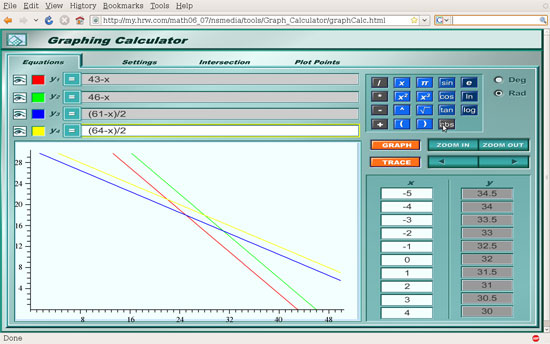SEARCH HOMEMath Central Quandaries & QueriesQuestion from Olivia, a student: Hi, I'm Olivia and I'm in 8th grade studying algebra 1. I'm having some trouble with my math project. It has to do with rules of stairway construction. It says that there are two generally accepted rules to building stairs. Rule 1: x+y=43 and x+y=46 (cm) Rule 2: 2y+x=61 and 2y+x=64 (y is the riser and x is the tread length) I cant figure out how to graph them...it says they're supposed to intersect to make a square which is like the safety zone for building stairs (the stairs' rise and tread lengths are plotted as points) any points outside of that square are considered unsafe. Did i explain it ok? Thanks for your help. --oliviaHI Olivia.

I first converted each of the four equations into y = form, then I used an online graphing calculator here http://my.hrw.com/math06_07/nsmedia/tools/Graph_Calculator/graphCalc.html to draw these lines.The middle quadrilateral is the "safety zone". It isn't square as you see.

The region between the red and green lines obeys Rule 1 and the area between the blue and yellow lines obeys Rule 2. So you can see that only the parallelogram region obeys both rules at the same time.

Hope this helps,
Stephen La Rocque.Math Central is supported by the University of Regina and The Pacific Institute for the Mathematical Sciences.